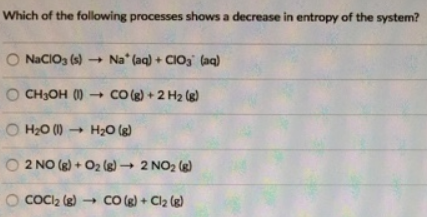# Problem: Which of the following processes shows a decrease in entropy of the system? NaClO3 (s) → Na+ (aq) ClO3- (aq) CH3OH (l) → CO (g) + 2H 2 (g) H2O(l) → H2O (g) 2NO (g) + O2 (g) → 2NO2 (g) COCl2 (g) → CO (g) + Cl 2 (g)

###### FREE Expert Solution
96% (441 ratings)###### Problem Details

Which of the following processes shows a decrease in entropy of the system?

NaClO3 (s) → Na+ (aq) ClO3- (aq)

CH3OH (l) → CO (g) + 2H 2 (g)

H2O(l) → H2O (g)

2NO (g) + O2 (g) → 2NO2 (g)

COCl2 (g) → CO (g) + Cl 2 (g)